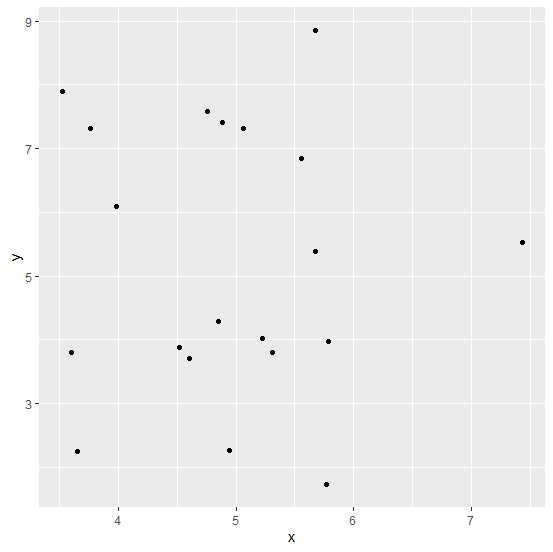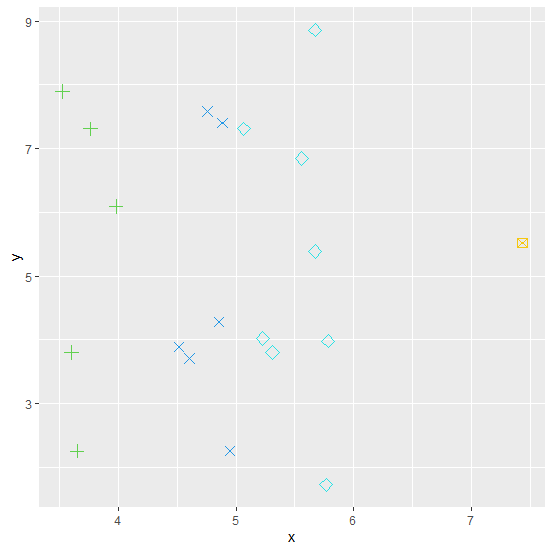# How to create a scatterplot using ggplot2 with different shape and color of points based on a variable in R?

In general, the default shape of points in a scatterplot is circular but it can be changed to other shapes using integers or sequence or the variable. We just need to use the argument shape inside geom_point function and pass the variable name. For example, if we want to create the scatterplot with varying shapes of a variable x then we can use geom_point(shape=x). And if we want to change the size then integer values can be used.

## Example

Consider the below data frame −

Live Demo

set.seed(151)
x<-rnorm(20,5,1)
y<-rnorm(20,5,2)
df<-data.frame(x,y)
df

## Output

      x       y
1 4.948461 2.255857
2 5.765737 1.726474
3 4.853260 4.280697
4 4.886814 7.402230
5 4.604489 3.708252
6 5.782276 3.978782
7 3.602522 3.801754
8 3.981162 6.091206
9 5.229476 4.017412
10 5.672173 5.383071
11 4.515448 3.882945
12 5.560609 6.845399
13 5.066156 7.307996
14 3.650124 2.255179
15 4.757084 7.580363
16 3.763259 7.309804
17 3.525322 7.891359
18 7.437159 5.522026
19 5.673526 8.858292
20 5.310040 3.800228

Loading ggplot2 package and creating point chart between x and y −

## Example

library(ggplot2)
ggplot(df,aes(x,y))+geom_point()

## OutputCreating the scatterplot by different shape of the points and the size = 3 −

## Example

ggplot(df,aes(x,y))+geom_point(shape=x,color=x,size=3)

## Output